Type a math problemalgebra
trigonometry
statistics
calculus
matrices
variables
list
AC
log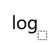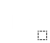ln
(
)
7
8
9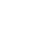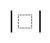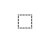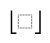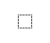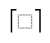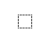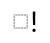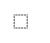τ
π
4
5
6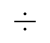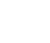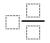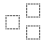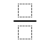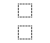%
θ
1
2
3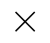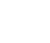<
>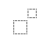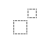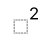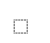x
i
0
.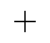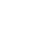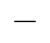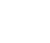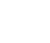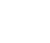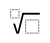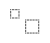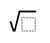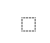y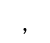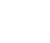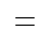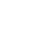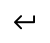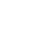Type a math problemalgebra
trigonometry
statistics
calculus
matrices
variables
list
AC
logln
(
)
7
8
9τ
π
4
5
6%
θ
1
2
3<
>x
i
0
.y